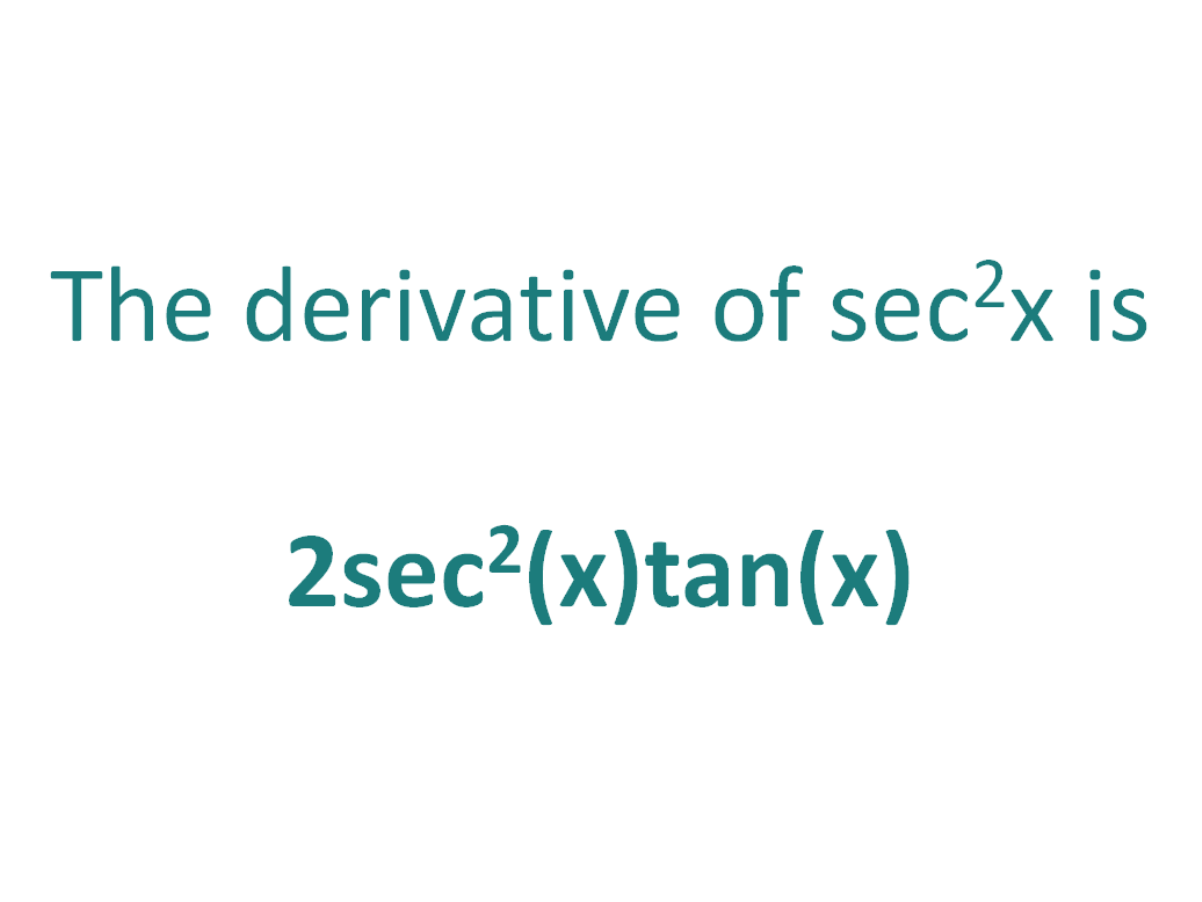# The Derivative of sec^2xThe derivative of sec^2x is 2.sec^2(x).tan(x)

## How to calculate the derivative of sec^2x

There are two methods that can be used for calculating the derivative of sec^2x.

The first method is by using the product rule for derivatives (since sec2(x) can be written as sec(x).sec(x)).

The second method is by using the chain rule for differentiation.

### Finding the derivative of sec^2x using the product rule

The product rule for differentiation states that the derivative of f(x).g(x) is f’(x)g(x) + f(x).g’(x)

The Product Rule:
For two differentiable functions f(x) and g(x)

If F(x) = f(x).g(x)

Then the derivative of F(x) is F'(x) = f’(x)g(x) + f(x)g'(x)

First, let F(x) = sec2(x)

Then remember that sec2(x) is equal to sec(x).sec(x)

So F(x) = sec(x)sec(x)

By setting f(x) and g(x) as sec(x) means that F(x) = f(x).g(x) and we can apply the product rule to find F'(x)

Using the product rule, the derivative of sec^2x is 2sec^2(x)tan(x)

### Finding the derivative of sec^2x using the chain rule

The chain rule is useful for finding the derivative of a function which could have been differentiated had it been in x, but it is in the form of another expression which could also be differentiated if it stood on its own.

In this case:

• We know how to differentiate sec(x) (the answer is sec(x)tan(x))
• We know how to differentiate x2 (the answer is 2x)

This means the chain rule will allow us to perform the differentiation of the expression sec^2x.

#### Using the chain rule to find the derivative of sec^2x

Although the expression sec2x contains no parenthesis, we can still view it as a composite function (a function of a function).

We can write sec2x as (sec(x))2.

Now the function is in the form of x2, except it does not have x as the base, instead it has another function of x (sec(x)) as the base.

Let’s call the function of the base g(x), which means:

g(x) = sec(x)

From this it follows that:

sec(x)2 = g(x)2

So if the function f(x) = x2 and the function g(x) = sec(x), then the function (sec(x))2 can be written as a composite function.

f(x) = x2

f(g(x)) = g(x)2 (but g(x) = sec(x))

f(g(x)) = (sec(x))2

Let’s define this composite function as F(x):

F(x) = f(g(x)) = (sec(x))2

We can find the derivative of sec^2x (F'(x)) by making use of the chain rule.

The Chain Rule:
For two differentiable functions f(x) and g(x)

If F(x) = f(g(x))

Then the derivative of F(x) is F'(x) = f’(g(x)).g’(x)

Now we can just plug f(x) and g(x) into the chain rule.

How to find the derivative of sec^2x using the Chain Rule:

Using the chain rule, the derivative of sec^2x is 2.sec^2(x).tan(x)

Finally, just a note on syntax and notation: sec^2x is sometimes written in the forms below (with the derivative as per the calculations above). Just be aware that not all of the forms below are mathematically correct.

## The Second Derivative Of sec^2x

To calculate the second derivative of a function, differentiate the first derivative.

From above, we found that the first derivative of sec^2x = 2sec2(x)tan(x). So to find the second derivative of sec^2x, we need to differentiate 2sec2(x)tan(x).

We can use the product and chain rules, and then simplify to find the derivative of 2sec2(x)tan(x) is 4sec2(x)tan2(x) + 2sec4(x)

The second derivative of sec^2x is 4sec2(x)tan2(x) + 2sec4(x)

## Interesting property of the derivative of sec^2x

It is interesting to note that the derivative of sec2(x) is equal to the derivative of tan2(x).

The derivative of:
> sec2x = 2.sec2(x).tan(x)
> tan2x = 2.sec2(x).tan(x)